# 梯度法

$\boldsymbol{\dot x} = f(\boldsymbol{x},\boldsymbol{u},t), \tag{1}$

$\boldsymbol x(t_0) = \boldsymbol{x}_0, \tag{2}$

$J(\boldsymbol u) = \Phi(\boldsymbol x_T,T) + \int_{T}^{t_0}L(\boldsymbol x, \boldsymbol u, t), \tag{3}$

$H(t,\boldsymbol{x},\boldsymbol{u},\boldsymbol{\lambda})=L(t,\boldsymbol{x},\boldsymbol{u}) + \boldsymbol{\lambda}^{\rm T}(t)f(\boldsymbol{x},\boldsymbol{u},t), \tag{4}$

$\nabla J(\boldsymbol{u^k}) = \frac{\partial H}{\partial \boldsymbol{u^k}}. \tag{5}$

$\boldsymbol{\dot \lambda} = -\frac{\partial H}{\partial \boldsymbol{x}}, \quad \boldsymbol{\lambda}(T) = \frac{\partial K}{\partial \boldsymbol{x}_T}, \tag{6}$ $\frac{\partial H}{\partial \boldsymbol{u}} = 0. \tag{7}$

# 算法步骤

1. 根据系统状态方程和目标函数建立哈密顿函数 $(4)$ 和伴随方程 $(5)$。
2. 猜测第 0 步的控制量 $\boldsymbol u^0(t)$ ，$k=0$ 。
3. 把 $\boldsymbol u^k(t)$ （在算法开始时，$k=0$）代入 $(1)$ 和 $(2)$ 中，进行正向积分，得到 $\boldsymbol{x}^k(t)$。
4. 根据伴随方程和末值条件 $(5)$ 反向积分，得到 $\boldsymbol{\lambda}^k(t)$。
5. 把 $\boldsymbol u^k(t)$ 、 $\boldsymbol{x}^k(t)$ 和 $\boldsymbol{\lambda}^k(t)$ 代入到 $(3)$ 求解性能指标 $J(\boldsymbol{u^k})$ 以及梯度 $\nabla J(\boldsymbol{u^k}) = \partial H / \partial \boldsymbol{u^k}$。
6. 确定步长参数 $K^k$ 以及修改量 $\Delta u^{k}(t) = -K^k \nabla J(\boldsymbol{u^k}) k$。
7. 更新控制量 $\boldsymbol{u}^{k+1}(t) = \boldsymbol{u}^{k}(t) + \Delta u^{k}(t)$。
8. 更新下一步 $k=k+1$ ，判断 $\bigtriangledown J(\boldsymbol{u^k})$ 小于设定值 $\varepsilon$。若满足要求，则退出循环；否则，重复第3步至第7步的迭代过程，直至满足终止条件。

# 详细解释

1）首先需要推导出哈密顿函数 $(4)$ 、伴随方程 $(5)$ 和梯度 $(6)$ 的表达式，为

\begin{aligned} &H = 0.5*(x^2+u^2) - \lambda (x^2+u), \\ &\boldsymbol{\dot \lambda} = -\frac{\partial H}{\partial x} = -x + 2\lambda x, \quad \lambda(1) = 1, \\ &\nabla J = \frac{\partial H}{\partial u} = u+\lambda. \end{aligned}

2）猜测第 0 步的控制量。因为 $\lambda(1) = 1$ ，要让 $\nabla J = u(1)+\lambda(1) = 0$，那么有 $u(1)=0$。不妨先设 $u^0(t)=0$。

u = -0.0*ones(1, t_segment);        % 控制量的初值猜测


3）正向积分求 $x(t)$。把 $u^0(t)=0$ 代入状态方程中，为$x^0 = -(x^0)^2$。随后进行积分，并结合初值 $x^0(0)=10$，求得

$x^0(t)=\frac{10}{1+10t}.$

% 1) 正向积分状态方程得到 x(t)
[Tx, X] = ode45(@(t,x) stateEq(t,x,u,Tu), [t0 tf], initx, options);
x = X;

% 状态方程
function dx = stateEq(t,x,u,Tu)
u = interp1(Tu,u,t);
dx = -x^2+u;
end


4）反向积分求 $\lambda(t)$。把 $x^0(t)=\frac{10}{1+10t}$ 代入伴随方程中，得到

$\dot \lambda^0(t) = \frac{20}{1+10t}\lambda^0 - \frac{10}{1+10t},$

$\lambda^0(t) = 0.5 \left[1 - \frac{(1+10t)^2}{121} \right].$

% 2) 反向积分协态方程得到 p(t)
% 注意这里的时间区间！它是反的！意思就是反向积分！
[Tp, P] = ode45(@(t,p) costateEq(t,p,x,Tx), [tf, t0], initp, options);
p = P;

% 因为求得的协态变量按照时间反序排列，为保证 x 和 p 在同一时间序列下
% 需要调整其顺序
p =interp1(Tp,p,Tx);

% 协态方程
function dp = costateEq(t,p,x,Tx)
x = interp1(Tx,x,t);
dp = -x + 2*p.*x;
end


5）计算梯度。将 $u^0(t)=0$ 和 $\lambda^0(t)=0.5 \left[1 - \frac{(1+10t)^2}{121} \right]$ 代入 $\nabla J = u+\lambda$ 中，得到

$\nabla J(u^0) = 0.5 \left[1 - \frac{(1+10t)^2}{121} \right].$

% 计算 dH/du，即目标泛函的梯度deltaH
H_Norm(i,1) = dH'*dH;       % 为后面判断是否满足终止条件做准备

% 用梯度法求解 dH/du 的表达式
u = interp1(Tu,u,Tx);
dH = p + u;
end


6）确定步长和修改量。在本算例中，将步长定为 $K=0.55$2，那么控制修改量为

$\Delta u^0(t) = - 0.275 \left[1 - \frac{(1+10t)^2}{121} \right].$

$\nabla J(u^0) = -\frac{1}{4}\int_{0}^{1} \left[1 - \frac{(1+10t)^2}{121} \right]^2 \text{d}t.$

% 更新控制量（梯度法）
% 计算目标函数的值
J(i,1) = fun(tf,x,Tx,u,Tu);
fprintf('The value of the objective function is %.4f \n',J(i,1))

% 如果梯度 dH/du < epsilon，则退出循环
if H_Norm(i,1) < eps
break;
else
% 否则，更新控制量，进入下一次迭代
u_old = u;
end

K = 0.55;
dH = interp1(tx,dH,tu);
u_new = u - K*dH;
end


7）更新控制量。按照 $u^1(t)=u^0(t)+\Delta u^0(t)$ 更新控制量，为

$u^1(t) = - 0.275 \left[1 - \frac{(1+10t)^2}{121} \right].$

8）循环迭代。按照上述步骤循环迭代3）—7）步，直至满足条件要求 H_Norm(i,1)<eps 或者迭代次数达到设定上限。

# 代码

• 把步长改为了变步长，使用一维线搜索技术确定步长。使用armijo准则来搜索步长。
• 添加了共轭梯度法的最优控制解法，跟梯度法做个对比。
• control_update_by_gradient()为梯度法，control_update_by_conjugate_gradient()为共轭梯度法。
%--------------------------------------------------------------------------
% 说明：应用最速下降法求解最优控制问题
% 例子：《最优化与最优控制》 pp. 257 例13.1
% 类型：无控制约束的最优控制问题
% 时间：2022/06/25
%--------------------------------------------------------------------------
clear;clc;close all;

%% 主函数部分
eps = 1e-3;
options = odeset('RelTol', 1e-4, 'AbsTol',1e-4);
t0 = 0; tf = 1;
t_segment = 50;
Tu = linspace(t0, tf, t_segment);	% 将时间离散化
u = -0.0*ones(1, t_segment);        % 控制量的初值猜测
initx = 10;                         % 状态变量初值
initp = 0.1;                          % 协态变量初值
max_iteration = 50;                 % 最大迭代次数
H_Norm = zeros(max_iteration,1);
step = 0.55*ones(max_iteration,1);
J = zeros(max_iteration,1);
d = zeros(max_iteration,max_iteration);

%% 算法开始
tic;
for i = 1:max_iteration
disp('---------------------------');
fprintf('%d iterarion times \n',i)
% 1) 正向积分状态方程得到 x(t)
[Tx, X] = ode45(@(t,x) stateEq(t,x,u,Tu), [t0 tf], initx, options);

% 2) 反向积分协态方程得到 p(t)
x = X;
[Tp, P] = ode45(@(t,p) costateEq(t,p,x,Tx), [tf, t0], initp, options);
p = P;

% 因为求得的协态变量按照时间反序排列，为保证 x 和 p 在同一时间序列下
% 需要调整其顺序
p =interp1(Tp,p,Tx);

% 计算 dH/du，即目标泛函的梯度deltaH
H_Norm(i,1) = dH'*dH;

% 计算目标函数的值
J(i,1) = fun(tf,x,Tx,u,Tu);
fprintf('The value of the objective function is %.4f \n',J(i,1))

% 如果梯度 dH/du < epsilon，则退出循环
if H_Norm(i,1) < eps
% 显示最后一次的目标函数值
%         fprintf('The value of the objective function is %.4f \n',J(i,1))
break;
else
% 否则，更新控制量，进入下一次迭代
u_old = u;
% 计算更新步长
% 我发现步长的影响更大，使不使用armijo线搜索来搜索步长对结果影响不大（beta = 0.55）
% step = 0.1*ones(max_iteration,1) 时，用梯度法更新 u 的迭代次数为 39 次，共轭梯度法为 14 次
% step = 0.55*ones(max_iteration,1) 时，用梯度法更新 u 的迭代次数为 5 次，共轭梯度法为 6 次
% 而step = 0*ones(max_iteration,1) 时，用armijo线搜索来搜索步长，
% 则梯度法更新 u 的迭代次数为 6 次，共轭梯度法为 13 次
%         step(i,1) = armijo(u_old, -dH, tf, x, Tx, Tu);
end
end

if i == max_iteration
disp('---------------------------');
disp('已达最大迭代次数，停止算法.');
end
toc;

%% 画图
window_width = 500;
window_height = 416;
figure('color',[1 1 1],'position',[300,200,window_width,window_height]);
plot(Tx, X, 'x-','LineWidth',1.5);hold on;
plot(Tu, u, 'x-','LineWidth',1.5);
xlabel('Time');
ylabel('State & control');
set(gca,'FontSize',15,...
'FontName','Times New Roman',...
'LineWidth',1.5);
legend('$x(t)$','$u(t)$',...
'Location','Northeast',...
'FontSize',10,...
'interpreter','latex');

count = find(J==0);
if isempty(count)
t_plot = (1:length(J))';
J_plot = J;
else
if J(count(1)-1)-J(count(1)) > 1
t_plot = (1:count(1)-1)';
J_plot = J(1:count-1);
else
t_plot = (1:length(J))';
J_plot = J;
end
end
figure('color',[1 1 1],'position',[300+window_width,200,window_width,window_height]);
plot(t_plot,J_plot,'x-','LineWidth',1.5);
xlabel('Iterations');
ylabel('J');
set(gca,'FontSize',15,...
'FontName','Times New Roman',...
'LineWidth',1.5);
legend('$J$',...
'Location','Northeast',...
'FontSize',10,...
'interpreter','latex');

%% 子函数定义部分
% 状态方程
function dx = stateEq(t,x,u,Tu)
u = interp1(Tu,u,t);
dx = -x^2+u;
end

% 协态方程
function dp = costateEq(t,p,x,Tx)
x = interp1(Tx,x,t);
dp = -x + 2*p.*x;
end

% 用梯度法求解 dH/du 的表达式
u = interp1(Tu,u,Tx);
dH = p + u;
end

% 更新控制量（梯度法）
beta = 0.55;
dH = interp1(tx,dH,tu);
% 用armijo准则搜索步长
u_new = u - beta^step*dH;
% 不用armijo准则搜索步长
%     u_new = u - step*dH;
end

% 更新控制量（共轭梯度法）
beta = 0.55;
dH = interp1(tx,dH,tu);
if i == 1
d(:,i) = -dH;
else
beta = H_Norm(i)/H_Norm(i-1);
d(:,i) = -dH' + beta*d(:,i-1);
end
% 用armijo准则搜索步长
u_new = u + beta^step*d(:,i)';
% 不用armijo准则搜索步长
%     u_new = u + step*d(:,i)';

end

%--------------------------------------------------------------------------
% 说明：armijo准则非精确线搜索程序，用来求解迭代步长
% 文献：《最优化方法及其Matlab程序设计》 pp. 26
% 作者：袁亚湘
%--------------------------------------------------------------------------
function mk = armijo(xk, dk, tf, x, Tx, Tu)
dk = -interp1(Tx,dk,Tu);
beta = 0.55;
sigma = 0.5;
m = 0;
mmax = 20;
while m <= mmax
if fun(tf,x,Tx,xk+beta^m*dk,Tu) <= fun(tf,x,Tx,xk+beta^m*dk,Tu)+sigma*beta^m*gfun(tf,xk,Tu)*dk'
m = m+1;
break;
end
m = m+1;
end
mk = m;
% 这个 end 归属于 function
end

function J = fun(tf,x,Tx,u,Tu)
J = tf*(((x')*x)/length(Tx) + (u*u')/length(Tu));
end

function gJ = gfun(tf,u,Tu)
gJ = tf*(2*u)/length(Tu);
end


# 结果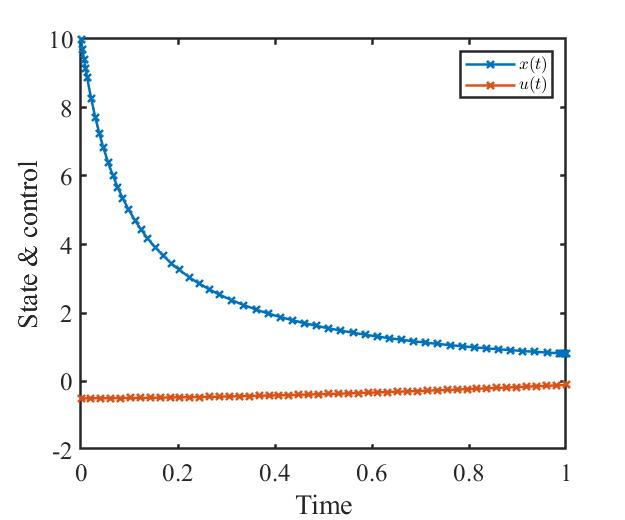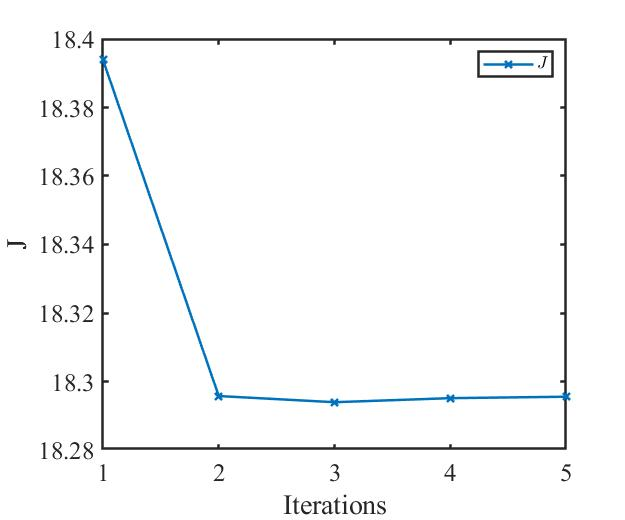# 对比

• 梯度法共轭梯度法对比；
• 不同步长条件下的两种算法结果对比；
• 是否使用armijo准则搜索步长的两种算法结果对比。

## 梯度法和共轭梯度法对比

u = -0.5*ones(1, t_segment);        % 控制量的初值猜测


step = 0.55*ones(max_iteration,1);
% 下面这一行代码在“算法开始”节中，记得注释
% step(i,1) = armijo(u_old, -dH, tf, x, Tx, Tu);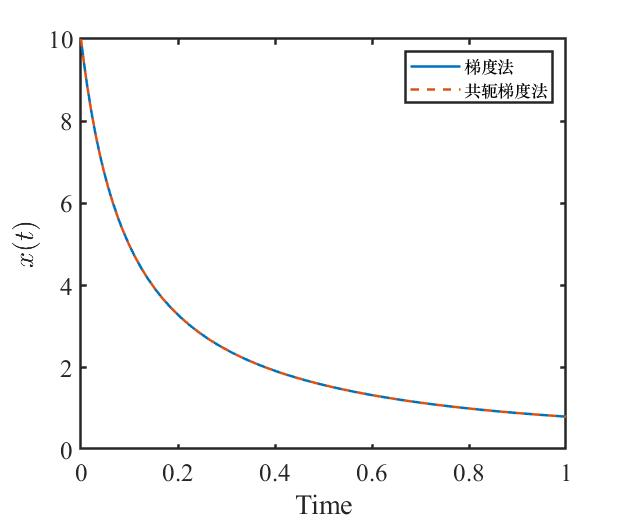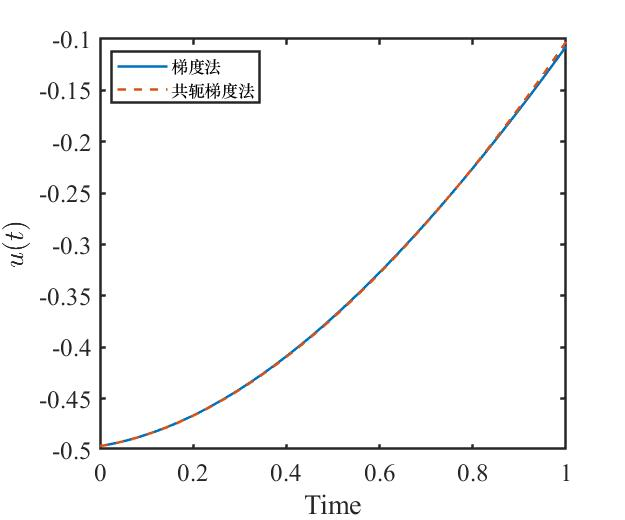## 不同步长条件下的两种算法结果对比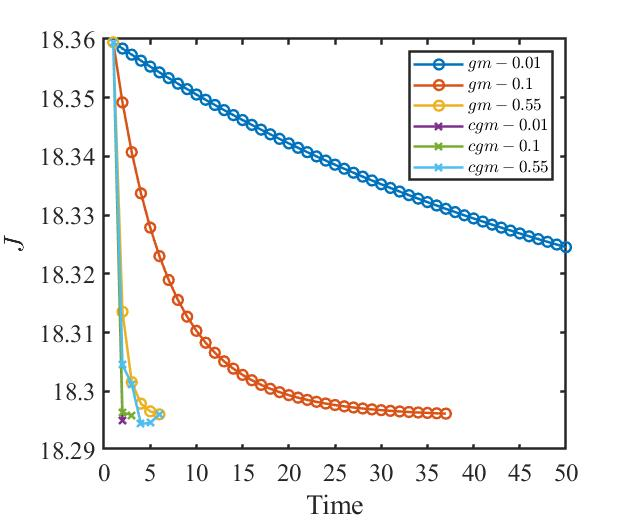## 是否使用armijo准则搜索步长的两种算法结果对比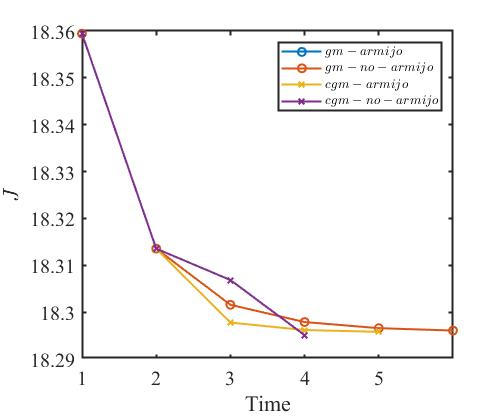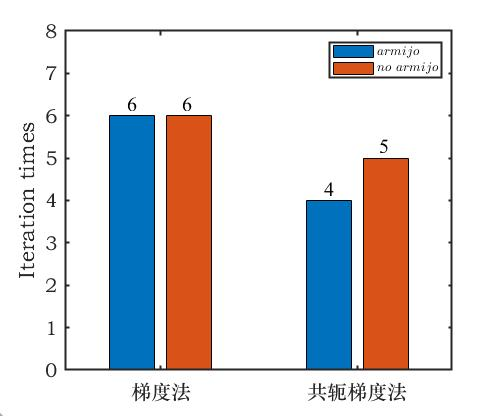# 思考

1. 如果想要详细了解推导过程，请见《最优化与最优控制》第2版第255页。

2. 步长不一定要取 $k=0.55$ ，根据不同的题目可以设置不同的步长，关键是要保证 $H$ 的值在逐渐下降。

3. 由负梯度方向加上一个修正量得到。

4. 算法设置最大迭代次数为50次。